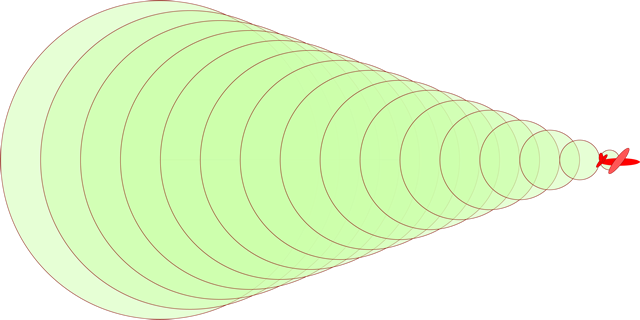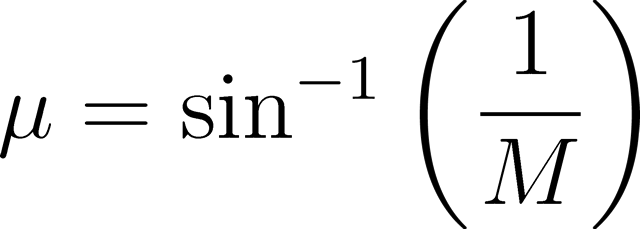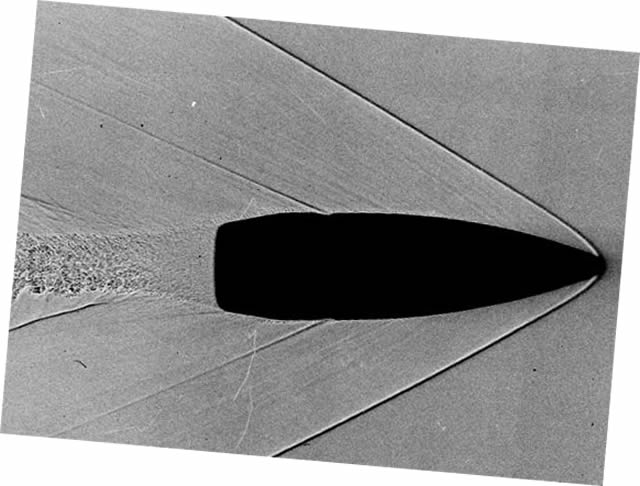# Sonic booms and Mach cones

When an object moves through the air it pushes the air in front of it away, creating a pressure wave. This pressure wave travels away from the object at the speed of sound. If the object itself is travelling at the speed of sound then these pressure waves build up on top of each other to create a shock wave, or sonic boom.On the left, an object travelling at Mach 0.7. On the right, an object travelling at Mach 1.0.

One of the most common misconceptions about sonic booms is that they occur only as an object accelerates beyond Mach 1.0 (through the “sound barrier”) and not during supersonic flight. This is not the case. A sonic boom is continuously created as long as an object is travelling faster than the speed of sound, and this sonic boom trails behind the object, creating a Mach cone.The shape of this Mach cone depends on the speed of the object, and the faster an object is, the narrower its Mach cone. For a supersonic or hypersonic object these cones can become very narrow and thus when the sonic boom from a high-speed aircraft reaches the ground the aircraft in question has already passed by and can be quite a distance away.

The angle of the Mach cone (μ) between the shock wave and the horizontal is simple to calculate from the object’s Mach number (M).For an F-22 Raptor travelling at its maximum speed of Mach 1.82 the angle of the Mach cone formed is 33.3°. If the Raptor was flying at an altitude of one hundred metres then it would always be sixty-five metres away (horizontally, in the direction of travel) from where its sonic boom was detected and 120 metres in a straight line from the point of impact. At a distance of 120 metres a person on the ground is hearing (or rather, feeling) the sonic boom 350 milliseconds after it is emitted, assuming the speed of sound is 340 metres per second.

By looking at Schlieren photographs, which can image differences in gas density, we can see the shockwave created, find the Mach cone angle, and from that calculate the speed of an object moving through the gas.In the photograph above the Mach cone angle is 28° and therefore the bullet must have been travelling at Mach 2.1 or 720 metres per second (assuming the speed of sound is 340 m/s).